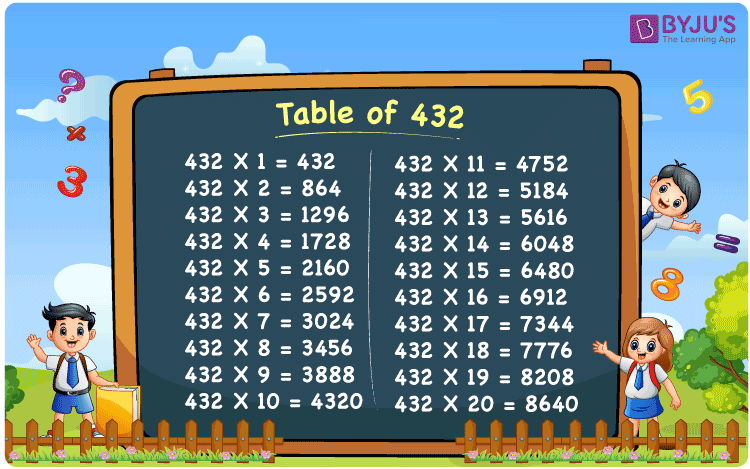Checkout JEE MAINS 2022 Question Paper Analysis : Checkout JEE MAINS 2022 Question Paper Analysis :

# Table of 432

The Multiplication Table for 432 is nothing more than the repetitive addition of the number 432. Repetitive addition is adding the same number multiple times. For example, adding 3 times 432, which is 432 + 432 + 432 is the same as 432 x 3 and that equals 1296. But adding the same number multiple times, say 8 times, can result in mistakes. Instead, the below-given chart on Table 432 can be referred to. For any large number multiplication table, use the Multiplication Calculator.

## Table of 432 Chart## What is the 432 Times Table?

In the table of 432, we are repeatedly adding 432 multiple times. And the table below gives a repetitive addition 10 times for the number 432.

 432 × 1 = 432 432 432 × 2 = 864 432 + 432 = 864 432 × 3 = 1296 432 + 432 + 432 = 1296 432 × 4 = 1728 432 + 432 + 432 + 432 = 1728 432 × 5 = 2160 432 + 432 + 432 + 432 + 432 = 2160 432 × 6 = 2592 432 + 432 + 432 + 432 + 432 + 432 = 2592 432 × 7 = 3024 432 + 432 + 432 + 432 + 432 + 432 + 432 = 3024 432 × 8 = 3456 432 + 432 + 432 + 432 + 432 + 432 + 432 + 432 = 3456 432 × 9 = 3888 432 + 432 + 432 + 432 + 432 + 432 + 432 + 432 + 432 = 3888 432 × 10 = 4320 432 + 432 + 432 + 432 + 432 + 432 + 432 + 432 + 432 + 432 = 4320

## Multiplication Table of 432

Given below is the multiplication table of 432 being done 20 times. The below table helps you with multiplication problems that require the tables of 432.

 432 × 1 = 432 432 × 2 = 864 432 × 3 = 1296 432 × 4 = 1728 432 × 5 = 2160 432 × 6 = 2592 432 × 7 = 3024 432 × 8 = 3456 432 × 9 = 3888 432 × 10 = 4320 432 × 11 = 4752 432 × 12 = 5184 432 × 13 = 5616 432 × 14 = 6048 432 × 15 = 6480 432 × 16 = 6912 432 × 17 = 7344 432 × 18 = 7776 432 × 19 = 8208 432 × 20 = 8640

## Solved Example on Table of 432

Example:

Solve for y:

432 y  = 7000 + 776

Solution: y = 18

432 y = 7000 + 776

432 y = 7776

y = 7776 / 432

y = 18.

## Frequently Asked Questions on Table of 432

### Question 1: What is 19 times 432?

Answer: 19 times 432 is 8208.